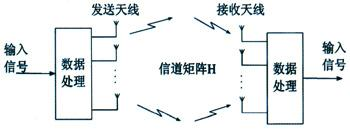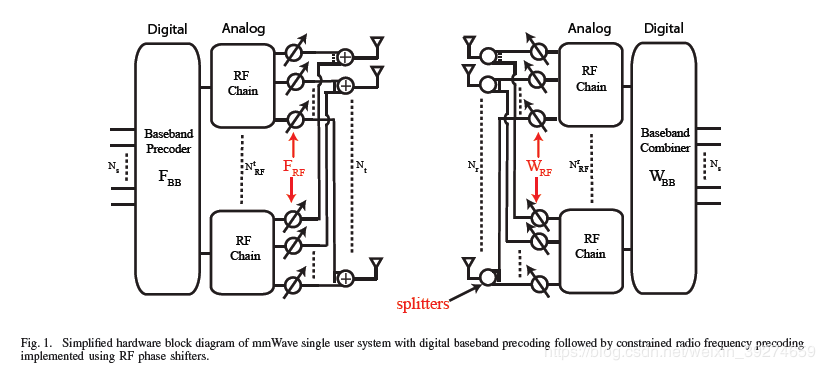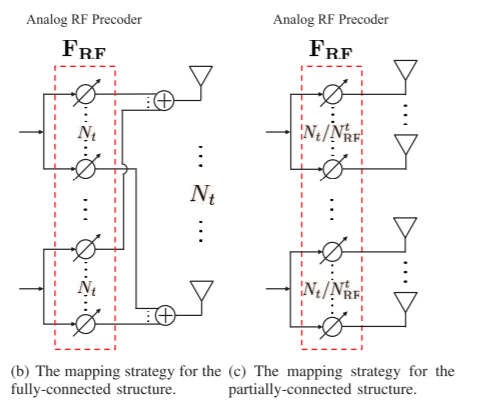# 系统模型与数学建模• 发送端的DAC （数字信号转模拟信号）
• 发送端的上变频操作
• 接收端的ADC（模拟信号转数字信号）
• 接收端的下变频操作• N s N_\mathrm{s} : 发送信号的维度，也可以称为 同一时刻发送了 N s N_\mathrm{s} 路信号。

• N t N_\mathrm{t} , N r N_\mathrm{r} ： 发送与接收天线数

• N R F t N^t_\mathrm{RF} , N R F r N^r_\mathrm{RF} ：发送与接收的RF chain数目

• F B B \mathbf{F}_\mathrm{BB} , W B B \mathbf{W}_\mathrm{BB} ：发送和接收 数字波束成形矩阵

• F R F \mathbf{F}_\mathrm{RF} , W R F \mathbf{W}_\mathrm{RF} ：发送和接收 模拟波束成形矩阵
值得一提的是，很多论文中将发送波束成形矩阵称为预编码矩阵 (precoder)， 接收称为(combiner)，如果看到的话知道这是一回事就可以了。
这张图其实已经反映了HBF的核心特征：大大降低了RF chain的数目，从而节约了硬件开销。需要重点注意的是模拟波束成形和数字波束成形的区别。为了降低RF chain，后续的模拟波束成形是在模拟域（射频）上操作的，因此，主流结构使用相移器 (phase shifter)来实现。其优点是成本较低，缺点在于只能改变信号的相位。
有一些基本的关系：首先，要发送 N s N_\mathrm{s} 路信号，至少需要 N R F t ≥ N s N^t_\mathrm{RF}\ge N_\mathrm{s} 路射频链路。同时，为减轻硬件开销，往往有 N R F t < < N t N^t_\mathrm{RF}<< N_\mathrm{t} 。通过数学建模的方式，把整个运作流程给大家过一遍：

• N s × 1 N_\mathrm{s} \times 1 的发送向量 s \mathbf{s} , 经过数字域的波束成形(线性变换, 可表示为维度为 N R F t × N s N^t_\mathrm{RF} \times N_\mathrm{s} 的矩阵 F B B \mathbf{F}_\mathrm{BB} , 得到维度为 N R F t × 1 N^t_\mathrm{RF} \times 1 的向量。

• 接下来，由相移器实现的模拟波束成形矩阵 （建模为维度是 N t t × N R F N^t_\mathrm{t} \times N_\mathrm{RF} 的矩阵 F R F \mathbf{F}_\mathrm{RF} )对信号进行处理，得到发送天线上实际待发送的信号，维度为 N t t × 1 N^t_\mathrm{t} \times 1 的信号 x = F R F F B B s \mathbf{x}=\mathbf{F}_\mathrm{RF}\mathbf{F}_\mathrm{BB}\mathbf{s}

• 发送信号经过MIMO信道，可以将该线性变换建模为 N r × N t N_\mathrm{r} \times N_\mathrm{t} 的信道矩阵 H \mathbf{H} 。即接收天线上收到信号为 r = H x \mathbf{r} = \mathbf{H}\mathbf{x}

• 接收端会做一个和发送端镜像的操作，即先通过模拟波束成形后再进行数字波束成形。 最终得到的信号为 s ^ = W B B H W R F H r \hat{s}=\mathbf{W}_\mathbf{BB}^H\mathbf{W}_\mathbf{RF}^H\mathbf{r} 。这里，将接收端的操作写为 W R F H \mathbf{W}_\mathbf{RF}^H 而不是 W R F \mathbf{W}_\mathbf{RF} 只是一种主流的写法，其实两者都是可以的。当写为 W R F H \mathbf{W}_\mathbf{RF}^H 时， W R F \mathbf{W}_\mathbf{RF} 的维度是 N r × N R F N_\mathrm{r} \times N_\mathrm{RF}

s ^ = W B B H W R F H H F R F F B B s \hat{\mathbf{s}}=\mathbf{W}_\mathbf{BB}^H\mathbf{W}_\mathbf{RF}^H \mathbf{H}\mathbf{F}_\mathrm{RF}\mathbf{F}_\mathrm{BB}\mathbf{s}

s ^ = W H H F s . \hat{\mathbf{s}} = \mathbf{W}^H\mathbf{H}\mathbf{F}\mathbf{s}.

• 混合波束成形所需要设计的矩阵数目更多（共有4个）
• 由于硬件实现上的区别， F R F \mathbf{F}_\mathrm{RF} , W R F \mathbf{W}_\mathrm{RF} 是由相移器实现的，因此只能改变信号的相位，对应于数学建模后的限制为，矩阵的每一个元素的模为1. 这一限制也叫做恒模约束，是HBF设计问题中最棘手的限制之一。（改变信号的角度等价于信号乘以 e j θ e^{j\theta} , θ \theta 为改变的角度值）。
• 同时，对于宽带信道（如OFDM系统），由于 F R F \mathbf{F}_\mathrm{RF} , W R F \mathbf{W}_\mathrm{RF} 是在射频上实现，因此无法对每个子载波进行各自的模拟波束成形。相反，由于 F R F \mathbf{F}_\mathrm{RF} , W R F \mathbf{W}_\mathrm{RF} 是对经过RF chain后的时域信号进行线性变换，因此，对应到频域上是对每个子载波进行了相同的操作。也就是说，所有的子载波信号经过了同样的 F R F \mathbf{F}_\mathrm{RF} , W R F \mathbf{W}_\mathrm{RF} 处理。这个后面会再提到。为了便于理解，这篇文章考虑的是简单的窄带情形，也就不涉及这一问题。
• 尽管引入了许多限制，增大了波束成形的难度，但这样的结构大大降低了硬件开销（主要是减少了RF chain的数目）。

# 设计算法的思路

• 目标为 最小化 ∥ F o p t − F R F F B B ∥ F \left\|\mathbf{F}_{\mathrm{opt}}-\mathbf{F}_{\mathrm{RF}} \mathbf{F}_{\mathrm{BB}}\right\|_{F}
F o p t \mathbf{F}_{\mathrm{opt}} 表示全数字波束成形下的最优解。 由于在过去的几十年中，人们在传统小规模MIMO下的研究已有了较深的积累，因此已有无数成熟的算法来求解全数字波束成形下的最优解。而这一思路的优点可以概括为：站在前人的肩膀上，使用传统纯熟的算法求解出全数字最优解，然后设计HBF矩阵（即 F R F F B B \mathbf{F}_{\mathrm{RF}} \mathbf{F}_{\mathrm{BB}} ）使其尽可能的逼近该最优解。因此，数学建模为最小化两者差的F范数。
有许多文章使用了这一做法，较为经典的是这两篇文章。

• 直接作为一个全新的问题，不依赖于过去的算法，直接以性能指标为优化目标对四个矩阵进行优化。有两篇较为典型的文章。

# 一些仍然值得研究的问题

5G HBF的算法设计时至今日，也已有了多年的积累。本文所描述的系统模型和算法设计思路，基本已经被学者们做透了，然而，仍然有许多问题，值得进一步的探讨和思考

• 更进一步的硬件限制–部分连接结构。 本文描述的系统模型中的模拟波束成形结构，被称为fully-connected，即全连接结构。 这个结构需要 N t × N R F N_\mathrm{t}\times N_\mathrm{RF} 个相移器，这一开销也十分昂贵。因此， Alternating minimizatioalgorithms for hybrid precoding in millimeter wave MIMO systems中提出了一种partially-connected的结构，下图展示了两者的区别：左图中，每个RF chain和天线间都有一个相移器连接（这也是为什么我们可以把模拟波束成形操作建模为一个矩阵），而右图的partially-connected结构中，每根天线只与一个相移器相连。显然，这一结构会对问题的求解引入新的限制，即 F R F \mathbf{F}_\mathrm{RF} , W R F \mathbf{W}_\mathrm{RF} 需要满足是一个块对角矩阵。在实际中，partially-connected结构更节约硬件开销，更易于工程实现，因此该结构下的算法非常值得研究。

• 更进一步的硬件限制–有限精度的相移器。 在本文讨论的结构中，我们在设计 F R F \mathbf{F}_\mathrm{RF} , W R F \mathbf{W}_\mathrm{RF} 矩阵时只考虑了其只能改变信号相位的限制。而事实上，在实际中相移器所要改变信号角度的精度也是有限的 （本文所提到的文章中均假设了无限精度的相移器）。考虑实际的限制，那么 F R F \mathbf{F}_\mathrm{RF} , W R F \mathbf{W}_\mathrm{RF} 中的元素应该只能在一个离散的集合中选取。 一般我们将相移器按精度描述为n-bit 相移器，对应的就是有 2 n 2^n 种相位可以选择，即模拟波束成形矩阵的元素只能在 2 n 2^n 种相位中选择。

• 另一种硬件思路–有限精度ADC/DAC。这个其实和本文关系不大，也不是HBF的范畴了，但适用于大规模MIMO系统，因此简单提一下。其思路就是，全数字波束成形的缺点就在于RF chain太多，而RF chain太多的核心问题是数模转换器和模数转换器的成本太高。因此，这种思路是我不降低RF chain的数目，但每个RF chain只使用成本较低的有限精度ADC/DAC，以此来降低成本。

• 信道估计与信道信息。 本文所提到的论文中，都是假设了完美已知的信道信息，侧重于波束成形设计。但实际中，如何精确估计信道信息是一个非常重要的话题。同样，全数字结构下的信道估计方法不能直接套用到HBF结构下进行信道估计。而对于波束成形设计而言，能够对信道估计误差进行一些鲁棒性的设计，会更加难能可贵。

# 相关阅读10-22
07-255338
03-102万+
05-313210
05-044万+
03-155544
05-033万+
12-19328
05-111338
03-01
12-10629
05-023万+
02-293199
04-212258
©️2020 CSDN 皮肤主题: 数字20 设计师:CSDN官方博客